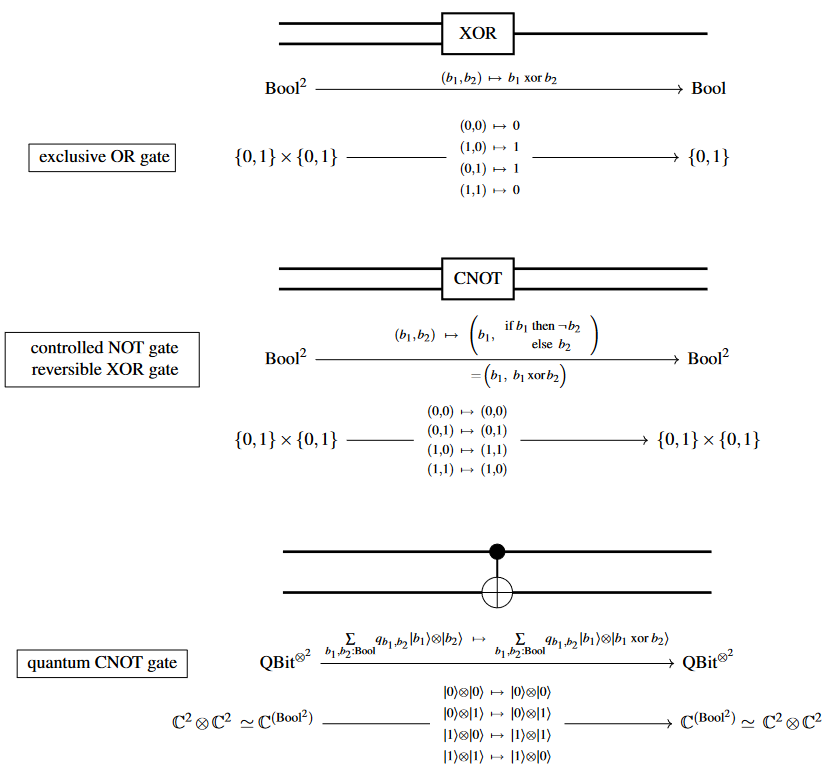# nLab CNOT gate

Contents

### Context

#### Computation

intuitionistic mathematics

# Contents

## Idea

The contolled NOT or reversible XOR-gate is the logic gate acting between pairs of bits which identically keeps the first bit in the pair and negates the second IFF the first was set.

This may be understood equivalently as

In quantum computing/quantum information theory, by the controlled NOT gate or CNOT gate one means the quantum logic gate which acts on a pair of qbits by the linear map which is given in this way on the canonical linear basis-elements of a pair of qbits:Similarly, by the CCNOT-gate (or Toffoli gate) one means the operation on triples of bits/qbit which keeps the first two and reverses the third iff the first two are both set.

Textbook accounts: